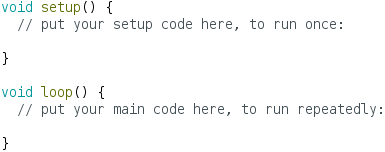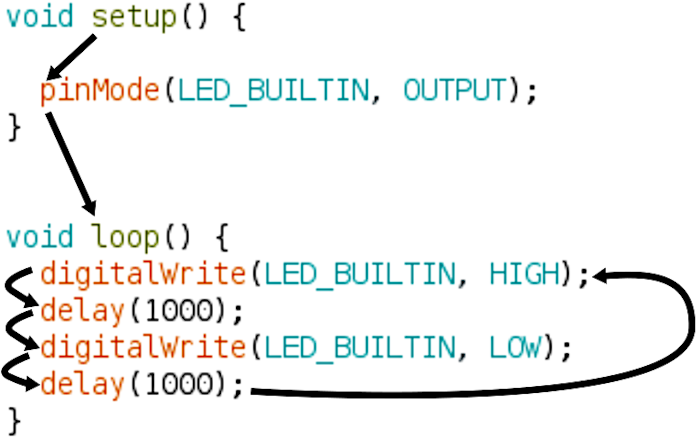Starting Electronics needs your help! Please make a donation to help cover our hosting and other costs. Click the donate button to send a donation of any amount.# How the Arduino Blink Sketch Works

Created on: 15 January 2022

How the Arduino Blink sketch works from the Arduino IDE example sketches. How to start to code or program for Arduino beginners and makers. This part of the Arduino tutorial for beginners explains the basics of the example sketch that blinks or flashes the on-board LED of an Arduino board. From this it can be seen how to start to write a new sketch for an Arduino. The following image shows the code from the Arduino blink sketch. As can be seen in the image, most of the code is explained in the comments.

Part 7 of the Arduino Tutorial for Beginners

## How Does the Arduino Blink Sketch Work?

Before looking at the Blink sketch code, examine the code from a new sketch. As can be seen in the following image in the next section, a new Arduino sketch consists of some basic code. This code is automatically inserted into a new Arduino sketch.

### Bare Minimum Arduino Sketch Code

Either click the New toolbar button in the Arduino IDE, or select File → New from the top Arduino IDE menu to open a new Arduino sketch. Alternatively, use the Ctrl + N keyboard shortcut. In addition to this, the same basic code is found by opening the BareMinimum sketch. That is, select File → Examples → 01.Basics → BareMinimum from the top menu bar to open this sketch.Bare Minimum Arduino Sketch Code that Helps Explain how the Blink Sketch Works

As the above image shows, there are two basic parts to any Arduino sketch. Specifically, these are the two functions called setup() and loop(). Each function is automatically called or run when loaded to an Arduino. Any code between the opening and closing braces of the setup() function runs only once. This occurs after powering up the Arduino or after resetting it. Typically, code that sets up hardware used later in the sketch is placed in the setup() function.

After code between the { opening brace, and } closing brace of setup() has finished running, code between the opening and closing brace of the loop() function is run. While setup() code runs only once, loop() code runs continually. That is, any lines of code between the opening and closing braces of loop() is run from top to bottom. After this the same code runs from top to bottom again. This is continually repeated.

### Arduino Blink Sketch Code Explanation

An Arduino sketch consists of a setup() function and a loop() function as is explained in the previous section. These functions of the Blink sketch example are explained below. Use the top menu bar of the Arduino IDE to open the Blink sketch. Select File → Examples → 01.Basics → Blink to open this sketch.

#### Arduino Blink Sketch setup() Function

The following image shows the setup() function of the Arduino Blink sketch example code.

##### Set Up an Arduino Pin as an Output

Because the Blink sketch controls the on-board LED of the Arduino, it must first set up the pin that this LED is connected to. Before the Arduino pin to which the LED is attached is able to drive or switch the LED, it must be set up as an output pin. Arduino digital pins are able to be set up as input pins or output pins. By default they are input pins. Call the pinMode() function to set up a pin as an output.

##### Call an Arduino Function and Pass Values to It

A function consists of a function name, followed by parentheses (). setup() and loop() are special functions that Arduino sketch code is placed into. These functions are special exceptions because code is placed between their opening and closing braces { and }. Most other functions are called in a sketch, such as the pinMode() function. Calling a function simply means using it in a sketch. As can be seen in the above code, the pinMode() function is called, simply by writing its name, followed by opening and closing parentheses, and finally a semicolon. Two values are passed to the pinMode() function, namely LED_BUILTIN and OUTPUT. These values or parameters are separated by a comma when passed to the function. They are passed to the function when they are placed between the function brackets or parentheses.

LED_BUILTIN is a special name that specifies the pin on an Arduino board to which the on-board LED is attached. OUTPUT specifies to the pinMode() function to set the pin up as an output pin.

##### Arduino Library Functions

The pinMode() function is what is known as an Arduino library function. Various functions are grouped together in a library and are available for a programmer to use in sketches. The Arduino reference contains information on the Arduino library functions.

The Arduino pinMode() function reference contains information on how to use this function. On this page is a description of the function, and its syntax. In addition, there is information on the parameters or values that are passed to this function.

#### Arduino Blink Sketch loop() Function

The following image shows the loop function of the Arduino Blink example sketch.

Four functions are called from within the Arduino Blink sketch loop() function block. As can be seen in the above image, digitalWrite() is called twice, and delay() is called twice.

##### Arduino digitalWrite() Function

The digitalWrite() function sets the voltage level on an Arduino pin that has been set up as an output. As can be seen in the code in the above image, digitalWrite is passed two values between parentheses and separated by a comma. LED_BUILTIN is the first parameter or value passed to digitalWrite(). This means that digitalWrite() will operate on the Arduino pin to which the on-board LED is attached. The second parameter passed to digitalWrite() is HIGH the first time that it is called. Subsequently it is called with this parameter set to LOW. HIGH causes digitalWrite to switch the on-board LED on, and LOW causes it to switch the LED off. This function is documented in the Arduino digitalWrite() language reference.

##### Arduino delay() Function

The Arduino delay() function causes a delay for a certain number of milliseconds, as described in the Arduino delay() language reference. In the Blink example sketch, delay() is passed a value of 1000. This results in delay() causing a 1000ms (one thousand millisecond) delay. 1000ms is the same as 1s, or one second. This delay leaves the on-board LED on for 1s. After this, it leaves the on-board LED off for 1s. Because the code that switches the on-board LED on and off is in the Arduino loop() function, it repeats over and over until the Arduino power is switched off. This causes the on-board LED to continually blink on and off.

## How the Arduino Blink Sketch Works Summary

The following image summarizes program flow of the Arduino Blink example sketch.The setup() function is automatically called when the Arduino board is powered up or reset. As a result, the pinMode() function in the setup() function is called or run. This causes the pin that the built-in LED is attached to, to be set up as an output pin.

The loop() function automatically runs after the setup() function. All of the lines of code in the loop() function run from top to bottom, as the lines at the left of the above image show. When the last function before the closing brace of loop() finishes running, execution of the first function at the top of the loop() function commences. The arrow at the right of the above image illustrates this. This completes the loop, and it can be seen why the function is called a loop.

From the above explanation it is shown that to start to program or write code for Arduino, it is necessary to start with a setup() and loop() function. Hardware is configured in the setup() part of the sketch. The main functionality of the sketch is placed in the loop() function.